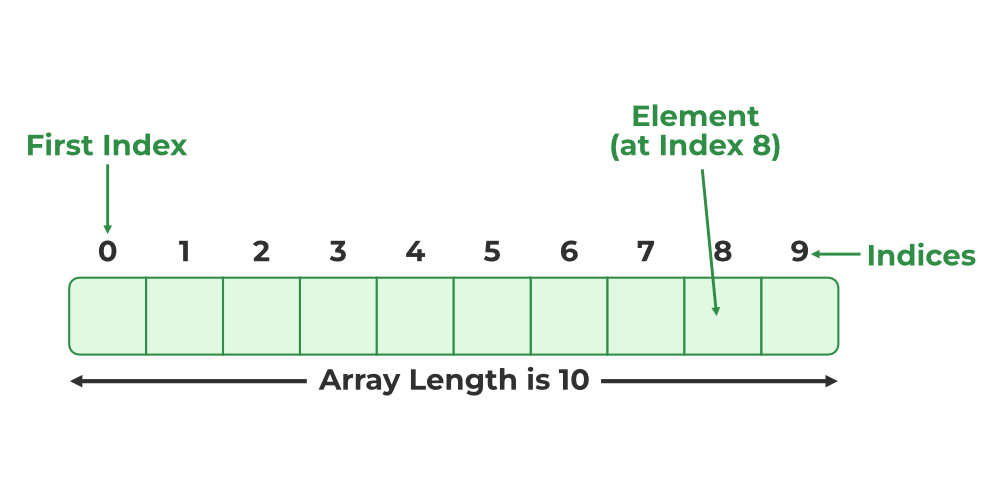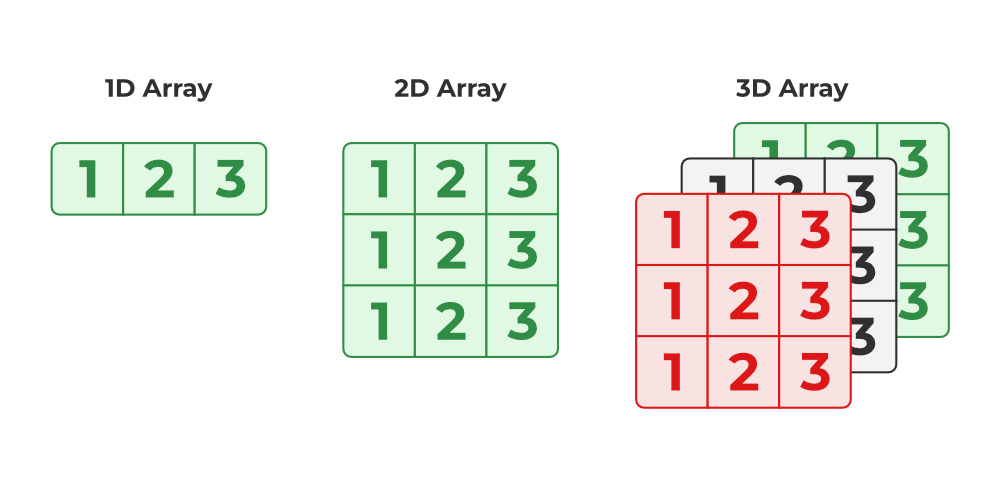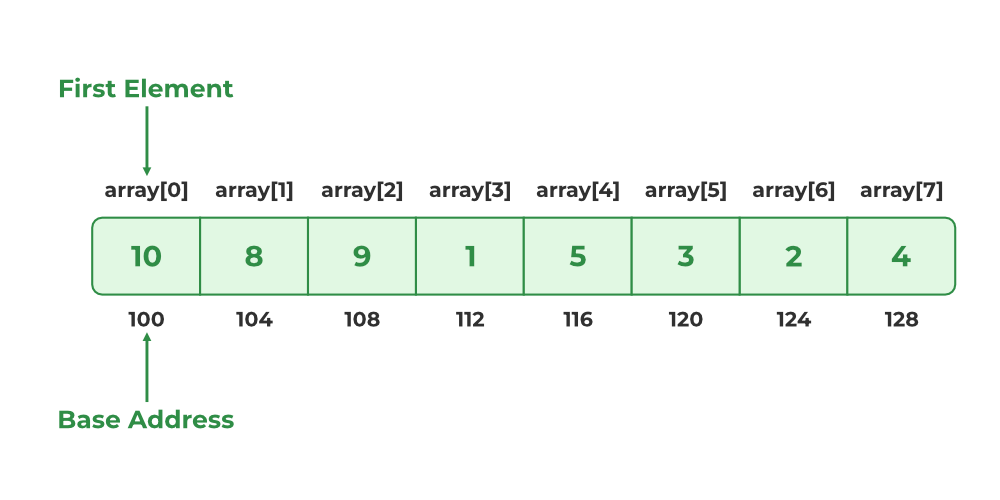# Properties of Array in C

An array in C is a fixed-size homogeneous collection of elements stored at a contiguous memory location. It is a derived data type in C that can store elements of different data types such as int, char, struct, etc. It is one of the most popular data types widely used by programmers to solve different problems not only in C but also in other languages.

The properties of the arrays depend on the programming language. In this article, we will study the different properties of Array in the C programming language.

1. Fixed Size Collection
2. Homogeneous Elements
3. Indexing in Array
4. Dimensions of Array
5. Contiguous Storage
6. Random Access
7. Array name relation with pointer
8. Bound Checking
9. Array Decay

## C Array Properties

### 1. Fixed Size of an Array

In C, the size of an array is fixed after its declaration. It should be known at the compile time and it cannot be modified later in the program. The below example demonstrates the fixed-size property of the array.

Example:

## C

 `// C Program to Illustrate the Fixed Size Properties of the` `// Array` `#include `   `int` `main()` `{`   `    ``// creating a new array of size 5` `    ``int` `array = { 1, 2, 3, 4, 5 };`   `    ``printf``(``"Size of Array Before: %d\n"``,` `           ``sizeof``(array) / ``sizeof``(``int``));`   `    ``// trying to increase the size of the array` `    ``array;` `    ``// not checking the size` `    ``printf``(``"Size of Array After: %d"``,` `           ``sizeof``(array) / ``sizeof``(``int``));`   `    ``return` `0;` `}`

Output

```Size of Array Before: 5
Size of Array After: 5```

### 2. Homogeneous Collection

An array in C cannot have elements of different data types. All the elements are of the same type.

Example:

## C

 `// C program to Demonstrate the Homogeneous Property of the` `// C Array` `#include `   `int` `main()` `{`   `    ``// declaring integer array` `    ``int` `arr = { 1, 2 };`   `    ``// trying to store string in the third element` `    ``arr = ``"Geeks"``;`   `  ``// printing elements` `    ``printf``(``"Array: %d\n"``, arr);` `    ``printf``(``"Array: %d\n"``, arr);` `    ``printf``(``"Array: %s"``, arr);`   `    ``return` `0;` `}`

Output

```main.c: In function ‘main’:
main.c:12:16: warning: assignment to ‘int’ from ‘char *’ makes integer from pointer without a cast [-Wint-conversion]
12 |         arr = "Geeks";
|                ^
main.c:17:28: warning: format ‘%s’ expects argument of type ‘char *’, but argument 2 has type ‘int’ [-Wformat=]
17 |         printf("Array: %s", arr);
|                           ~^   ~~~~~~
|                            |      |
|                            char * int
|                           %d
Array: 1
Array: 2```

### 3. Indexing in an Array

Indexing of elements in an Array in C starts with 0 instead of 1. It means that the index of the first element will be 0 and the last element will be (size – 1) where size is the size of the array.Indexing of Elements in C Array

Example:

## C

 `// C Program to Illustrate Array Indexing in C` `#include `   `int` `main()` `{`   `    ``// creating integer array with 2 elements` `    ``int` `arr = { 10, 20 };`   `    ``// printing element at index 1` `    ``printf``(``"Array: %d\n"``, arr);`   `    ``// printing element at index 0` `    ``printf``(``"Array: %d"``, arr);` `  `  `    ``return` `0;` `}`

Output

```Array: 20
Array: 10```

As we see in the above example, at index 1, the second element is present while at index 0, the first element is present.

### 4. Dimensions of the Array

An array in C can be a single dimensional like a 1-D array or multidimensional like a 2-D array, 3-D array, and so on. It can have any number of dimensions. The number of elements in a multidimensional array is the product of the size of all the dimensions.Arrays of Different Dimensions

Example:

## C

 `// C Program to create multidimensional array` `#include `   `int` `main()` `{`   `    ``// creating 2d array` `    ``int` `arr2d = { 1, 2, 3, 4 };`   `    ``// creating 3d array` `    ``int` `arr3d = { 1, 2, 3, 4, 5, 6, 7, 8 };`   `    ``printf``(``"2D Array: "``);` `    ``// printing 2d array` `    ``for` `(``int` `i = 0; i < 2; i++) {` `        ``for` `(``int` `j = 0; j < 2; j++) {` `            ``printf``(``"%d "``, arr2d[i][j]);` `        ``}` `    ``}`   `    ``printf``(``"\n3D Array: "``);` `    ``// printing 3d array` `    ``for` `(``int` `i = 0; i < 2; i++) {` `        ``for` `(``int` `j = 0; j < 2; j++) {` `            ``for` `(``int` `k = 0; k < 2; k++) {` `                ``printf``(``"%d "``, arr3d[i][j][k]);` `            ``}` `        ``}` `    ``}`   `    ``return` `0;` `}`

Output

```2D Array: 1 2 3 4
3D Array: 1 2 3 4 5 6 7 8 ```

### 5. Contiguous Storage

All the elements in an array are stored at contiguous or consecutive memory locations. We can easily imagine this concept in the case of a 1-D array but multidimensional arrays are also stored contiguously. It is possible by storing them in row-major or column-major order where the row after row or column after the column is stored in the memory. We can verify this property by using pointers.

Example:

## C++

 `// C Program to Verify the Contiguous Storage of Elements in` `// an Array` `#include `   `int` `main()` `{`   `    ``// creating an array of 5 elements` `    ``int` `arr = { 1, 2, 3, 4, 5 };`   `    ``// defining pointers to 2 consecutive elements` `    ``int``* ptr1 = &arr;` `    ``int``* ptr2 = &arr;`   `    ``// printing the address of arr and arr` `    ``printf``(``"Address of arr : %p\n"``, ptr1);` `    ``printf``(``"Address of arr : %p"``, ptr2);`   `    ``return` `0;` `}`

Output

```Address of arr : 0x7fffb8cc1ef4

In the above example, the difference between the addresses of arr and arr is 4 bytes which is the memory required to store a single integer. So, at memory addresses 0x7ffebc02e054 to 0x7ffebc02e057, arr is stored and in the next 4 bytes, arr is stored. The same is true for all the elements.

It is one of the defining properties of an Array in C. It means that we can randomly access any element in the array without touching any other element using its index. This property is the result of Contiguous Storage as a compiler deduces the address of the element at the given index by using the address of the first element and the index number.

`Address of ith = Address of 1st Element + (Index * Size of Each Element)`Array in C

We can verify this by using Pointer Arithmetic.

Example:

## C

 `// C Program to check the random access property of the` `// array` `#include `   `int` `main()` `{`   `    ``// creating an array of 5 elements` `    ``int` `arr = { 1, 2, 3, 4, 5 };`   `    ``// address of first element` `    ``int``* ptr = &arr;`   `    ``// printing arr` `    ``printf``(``"Array: %d\n"``, arr);`   `    ``// printing element at index 3 using ptr` `    ``printf``(``"Array using pointer to first element = %d"``,` `           ``*(ptr + 3));`   `    ``return` `0;` `}`

Output

```Array: 4
Array using pointer to first element = 4```

Note: We have not multiplied the size of each element in the code as the compiler deduce and multiply it automatically by itself whenever we perform pointer arithmetic.

### 7. Relationship between Array and Pointers

Arrays are closely related to pointers in the sense that we can do almost all the operations possible on an array using pointers. The array’s name itself is the pointer to its first element.

Example:

## C

 `// C Program to Illustrate the Relationship Between Array` `// and Pointers` `#include `   `int` `main()` `{`   `    ``// creating an array with 3 elements` `    ``int` `arr = { 1, 2, 3 };`   `    ``int``* ptr = &arr;`   `    ``// Pointer to first element` `    ``printf``(``"Pointer to First Element: %p\n"``, ptr);`   `    ``// Array name as pointer` `    ``printf``(``"Arran Name: %p"``, arr);`   `    ``return` `0;` `}`

Output

```Pointer to First Element: 0x7ffec5059660
Arran Name: 0x7ffec5059660```

The relationship between pointer and array is very deep and we can study more about it in other articles such as – Pointer to an Array | Array Pointer

### 8. Bound Checking

Bound checking is the process in which it is checked whether the referenced element is present within the declared range of the Array. In C language, array bound checking is not performed so we can refer to the elements outside the declared range of the array leading to unexpected errors.

Example:

## C

 `// C Program to Illustrate the Out of Bound access in arrays` `#include `   `int` `main()` `{`   `    ``// creating new array with 3 elements` `    ``int` `arr = { 1, 2, 3 };`   `    ``// trying to access out of bound element` `    ``printf``(``"Some Garbage Value: %d"``, arr);`   `    ``return` `0;` `}`

Output

`Some Garbage Value: 0`

As seen in the above example, there is no error shown by the compiler while accessing memory that is out of array bounds.

### 9. Array Decay

Array decay is the process in which an array in C loses its dimension in certain conditions and decays into pointers. After this, we cannot determine the size of the array using sizeof() operator. It happens when an array is passed as a pointer.

Example:

## C

 `// C Program to Demonstrate the Array Decay` `#include `   `// function` `void` `func(``int``* arr)` `{` `    ``printf``(``"Sizeof Value in Function: %d"``, ``sizeof``(arr));` `}`   `int` `main()` `{`   `    ``// creating array with 3 elements` `    ``char` `arr;`   `    ``printf``(``"Sizeof Value in Main: %d\n"``, ``sizeof``(arr));`   `    ``// passing array` `    ``func(arr);`   `    ``return` `0;` `}`

Output

```Sizeof Value in Main: 3
Sizeof Value in Function: 8```

The size of the array in the main() is 3 bytes which is the actual size of the array but when we check the size of the array in func(), the size comes out to be 8 bytes which instead of being the size of the array, it is the size of the pointer to the first element of the array.

Whether you're preparing for your first job interview or aiming to upskill in this ever-evolving tech landscape, GeeksforGeeks Courses are your key to success. We provide top-quality content at affordable prices, all geared towards accelerating your growth in a time-bound manner. Join the millions we've already empowered, and we're here to do the same for you. Don't miss out - check it out now!

Previous
Next